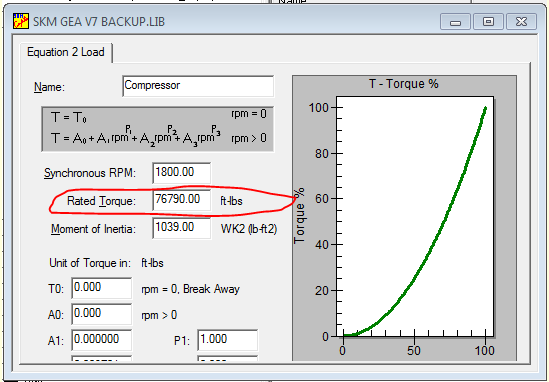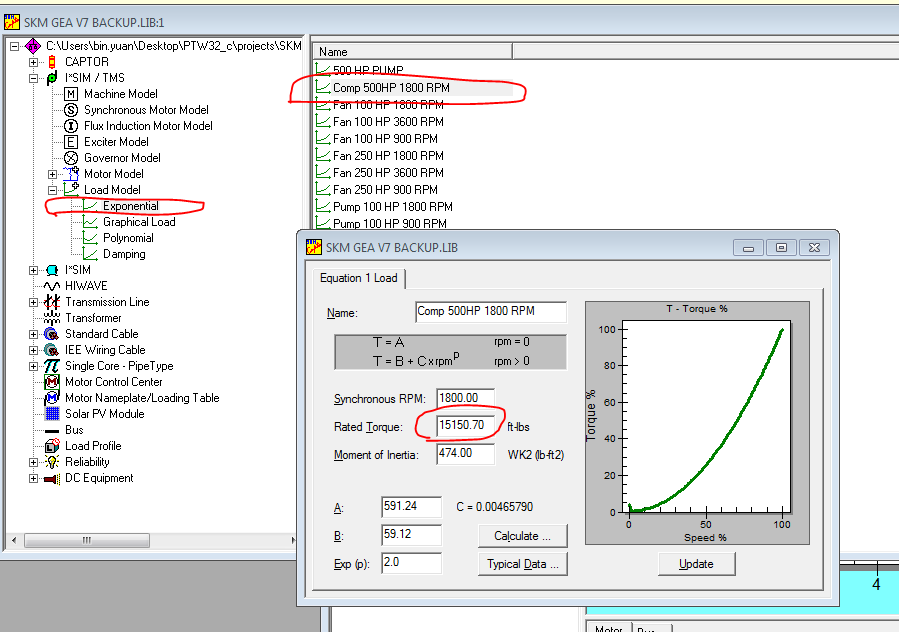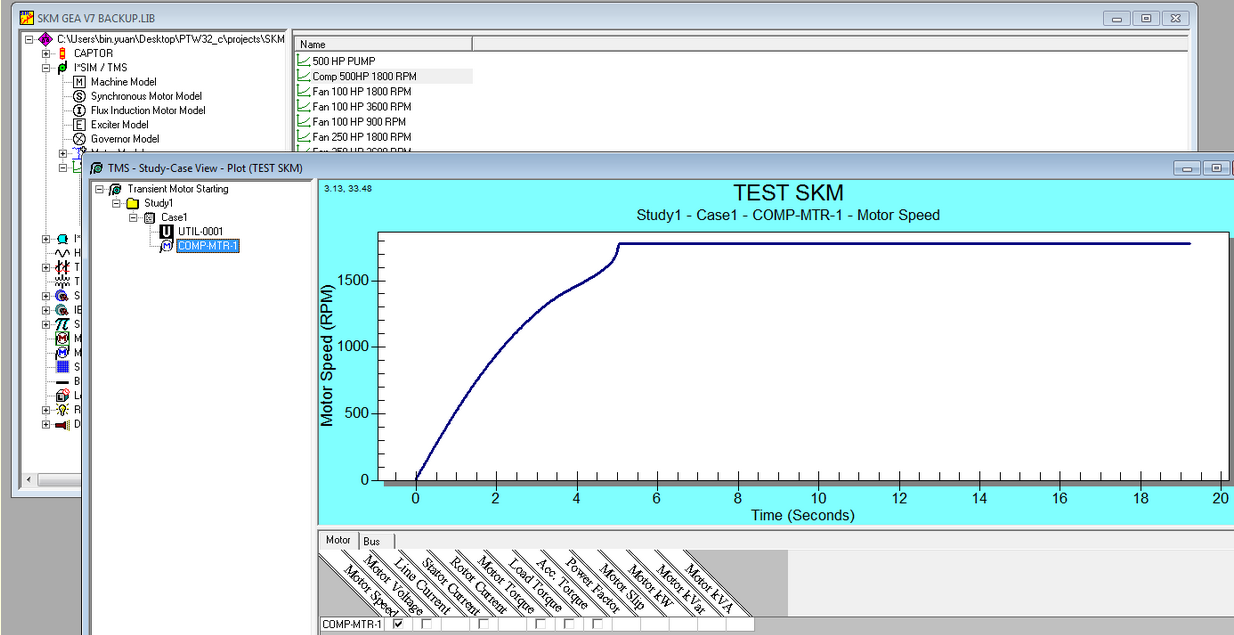• Sales
• Library Model Request
• Technical Support

# Motor unable to start in TMS

The problem occurred in user motor load model:

Scenario:
1. The rated torque is 76790, which is much bigger than motor rated torque.
2. The load torque curve is not good for motor torque curve. It means that at some speed points, the motor torque is smaller than the load torque.Since the motor size is 5800 Hp, we suggest using the exponential load model “Comp 500HP 1800rpm” and changing the rated torque to a value less than 17048 ft-lbs (motor torque base). For example, at 15150 ft-lbs, the motor will start normally.